ИЗДАЕТСЯ ПО БЛАГОСЛОВЕНИЮ ВЫСОКОПРЕОСВЯЩЕННЕЙШЕГО МИТРОПОЛИТА ТОБОЛЬСКОГО И ТЮМЕНСКОГО ДИМИТРИЯ
#!/usr/bin/perl # # PicsRandomizer Version 0.3 # This script produces three files with random pictures and the URLs you # specify in pics_rand_list.txt to include in shtml. # # Copyright (c) 2002 Alexander Uvizhev . All rights reserved. # This program is free software; you can redistribute it and/or # modify it under the same terms as Perl itself. # ######## CONFIGURATION ######################################################## #Path to a directory with your pictures (relatively to index.shtml). \$dir = "/pic"; #Path to pics_rand_list.txt (All paths below are relative to this script). \$list = "pics_rand_list.txt"; #Path to a directory with your pictures (relatively to index.shtml). #\$dir_root = "../htdocs/"; \$dir_root = "../www/"; #These files to include in your shtml (no need to create them). \$img = \$dir_root."pic/img1"; \$img = \$dir_root."pic/img2"; \$img = \$dir_root."pic/img3"; \$img = \$dir_root."pic/img4"; \$img = \$dir_root."pic/img5"; \$img = \$dir_root."pic/img6"; ################################################################################ open (FILE, "< \$list") or die ("Cannot open file \$list"); until (eof(FILE)) { \$line = readline *FILE; if (\$line =~ m/^\s*\n|^\s*#/) {next;} \$line =~ m/(\S+)\s(\S+)/; \$name[\$i] = \$1; \$url[\$i] = \$2; \$i++; } close FILE; #while (\$x == \$x || \$x == \$x || \$x == \$x) { while (\$x == \$x || \$x == \$x || \$x == \$x || \$x == \$x || \$x == \$x || \$x == \$x || \$x == \$x || \$x == \$x || \$x == \$x || \$x == \$x) { \$x = sprintf ("%u", rand \$#name+1); \$x = sprintf ("%u", rand \$#name+1); \$x = sprintf ("%u", rand \$#name+1); \$x = sprintf ("%u", rand \$#name+1); \$x = sprintf ("%u", rand \$#name+1); } #while (\$x == \$x || \$x == \$x || \$x == \$x) { while (\$x == \$x || \$x == \$x || \$x == \$x || \$x == \$x || \$x == \$x) { \$x = sprintf ("%u", rand \$#name+1); } for (\$i=0;\$i<6;\$i++) { open (CURRENT, "> \$img[\$i]") or die ("Cannot open file \$img[\$i]"); # print CURRENT ""; print CURRENT ""; close CURRENT } print "Content-type: text/html\n\n";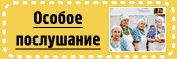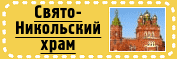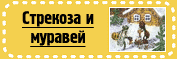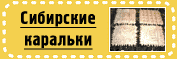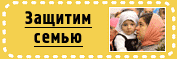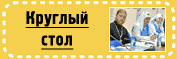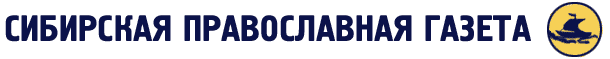### №10 2010 г.### Чудотворный образ Абалакской иконы Божией Матери будет доставлен в Новосибирскую, Томскую и Барнаульскую епархии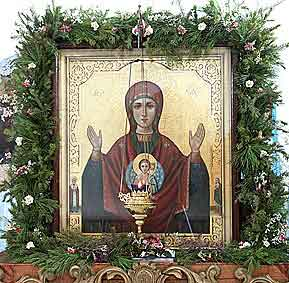21 сентября - великий праздник Рождества Пресвятой Богородицы. Во всех православных храмах епархии в этот день прошли праздничные Богослужения. Особенно радостным был праздник для прихожан и насель-ниц Богородице-Рождественского Ильинского женского монастыря города Тюмени.

Пребывание Абалакской святыни в Томской епархии приурочено к празднованию 200-летия храма в честь Абалакской иконы Божией Матери г. Томска.

Святыня посетит также Ишимское, Ялуторовское и Тюменское благочиния Тобольско-Тюменской епархии.

[ ФОРУМ ] [ ПОИСК ] [ ГОСТЕВАЯ КНИГА ] [ НОВОНАЧАЛЬНОМУ ] [ БОГОСЛОВСКОЕ ОБРАЗОВАНИЕ ]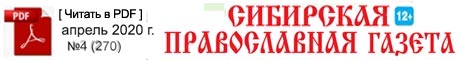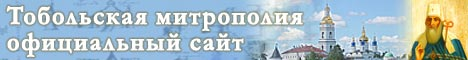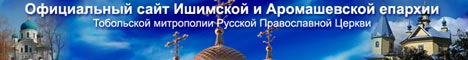Сибирская Православная газета 2020 г.